Author: Oscar Cronquist Article last updated on May 05, 2022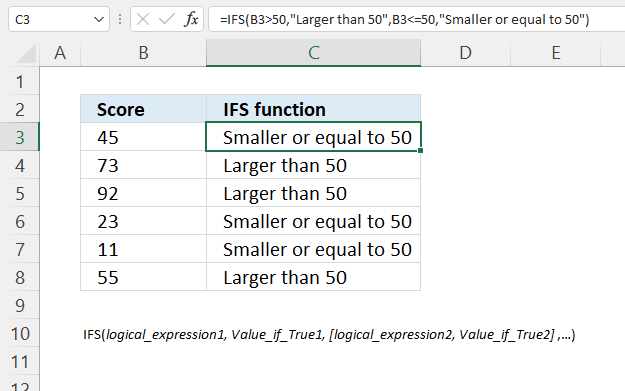The IFS function checks whether one or more conditions are met and returns a value that corresponds to the first TRUE condition.

The image above shows the IFS function in column C, it returns "Larger than 50" if the corresponding value on the same row in column B is larger than 50.

It also returns "Smaller than or equal to 50" if the corresponding value is smaller than or equal to 50.

## 1. IFS Function Syntax

IFS(logical_expression1, Value_if_True1, [logical_expression2, Value_if_True2],…)

The IFS function allows you to have up to 127 pairs of logical expressions and values if the logical expression evaluates to true.

## 2. IFS Function Arguments

 logical_expression1 Required. The logical expression you want to use. Value_if_True1 Required. The value to be displayed if the logical expression is met. [logical_expression2] Optional. Additional logical expression. [Value_if_True2] Optional. Additional value to be returned if the logical expression evaluates to true.

## 3. IFS function example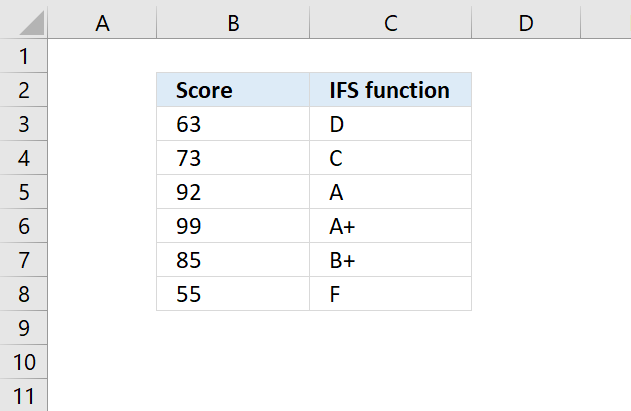The IFS function is a better alternative to nested IF functions, the formula becomes a lot simpler and easier to understand.

This example shows how to calculate a grade based on a score.

Formula in cell C3:

=IFS(B3>95, "A+", B3>89, "A", B3>84, "B+", B3>79, "B", B3>74, "C+", B3>69, "C", B3>64, "D+", B3>59, "D", B3>=0, "F")

The formula in the above picture calculates the grade based on the following score levels.

A+ score > 95
A score > 89.
B+ score > 84
B score > 79
C+ score > 74
C score > 69
D+ score > 64
D score > 59
F score > 60

## 4. IFS function alternativ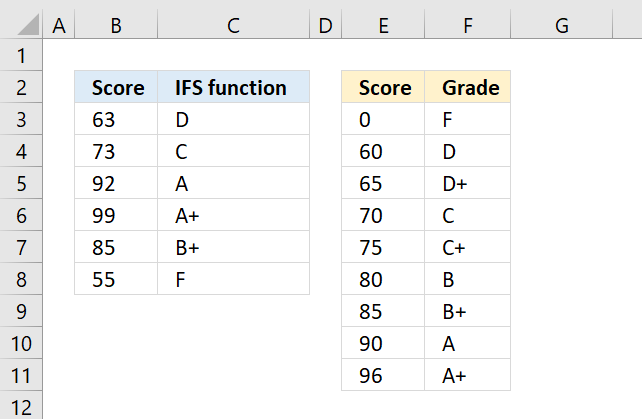The IFS function is an Excel 2016 function, however, you can replicate the behavior with the following formula if you have an earlier version of Excel.

Formula in cell C3:

=INDEX(\$F\$3:\$F\$11, MATCH(B3, \$E\$3:\$E\$11))

The formula above uses the table E3:F11 to match the score with the correct grade.

### 4.1 Explaining formula

#### Step 1 - Find a relative position in the table of a given value

The MATCH function returns the relative position of an item in an array or cell reference that matches a specified value in a specific order.

MATCH(lookup_value, lookup_array, [match_type])

MATCH(B3, \$E\$3:\$E\$11)

becomes

MATCH(63, {0; 60; 65; 70; 75; 80; 85; 90; 96})

Note, in this specific setup the MATCH function must have the numbers in the second argument sorted from smallest to largest.
The third argument determines what the requirements are, read more: MATCH function. I know there is no third argument in this example, however, the default value is 1 meaning: Finds the smallest value that is greater than or equal to lookup_value. Lookup_array must be sorted in a descending order

and returns 2.

#### Step 2 - Return the corresponding value

The INDEX function returns a value from a cell range, you specify which value based on a row and column number.

INDEX(array[row_num][column_num], [area_num])

INDEX(\$F\$3:\$F\$11, MATCH(B3, \$E\$3:\$E\$11))

becomes

INDEX({"F"; "D"; "D+"; "C"; "C+"; "B"; "B+"; "A"; "A+"}, 2)

and returns "D" in cell C3.

## 5. IFS function - convert month number to month name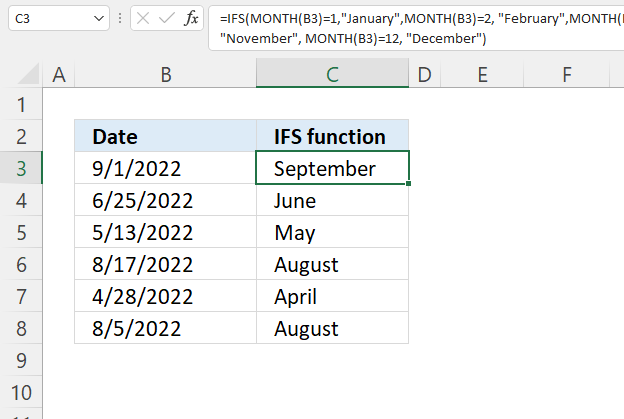Formula in cell C3:

=IFS(MONTH(B3)=1,"January",MONTH(B3)=2, "February",MONTH(B3)=3,"March",MONTH(B3)=4, "April", MONTH(B3)=5,"May",MONTH(B3)=6, "June", MONTH(B3)=7, "July", MONTH(B3)=8,"August",MONTH(B3)=9, "September",MONTH(B3)=10,"October",MONTH(B3)=11,"November", MONTH(B3)=12, "December")

### Explaining formula in cell B3

#### Step 1 - Calculate month number based on a date

The MONTH function extracts the month as a number from an Excel date.

MONTH(serial_number)

MONTH(B3)

becomes

MONTH(44805)

and returns 9.

#### Step 2 - Calculate logical expressions

The equal sign is a logical operator and lets you compare value to value in an Excel formula. The result is a boolean value TRUE or FALSE.

MONTH(B3)=1 evaluates to FALSE
MONTH(B3)=2 evaluates to FALSE
MONTH(B3)=3 evaluates to FALSE
MONTH(B3)=4 evaluates to FALSE
MONTH(B3)=5 evaluates to FALSE
MONTH(B3)=6 evaluates to FALSE
MONTH(B3)=7 evaluates to FALSE
MONTH(B3)=8 evaluates to FALSE
MONTH(B3)=9 evaluates to TRUE
MONTH(B3)=10 evaluates to FALSE
MONTH(B3)=11 evaluates to FALSE
MONTH(B3)=12 evaluates to FALSE

MONTH(B3)=9

becomes

9=9

and returns TRUE

#### Step 3 - Return the corresponding value

MONTH(B3)=1,"January",
MONTH(B3)=2, "February",
MONTH(B3)=3,"March",
MONTH(B3)=4, "April",
MONTH(B3)=5,"May",
MONTH(B3)=6, "June",
MONTH(B3)=7, "July",
MONTH(B3)=8,"August",
MONTH(B3)=9, "September",
MONTH(B3)=10, "October",
MONTH(B3)=11,"November",
MONTH(B3)=12, "December"

MONTH(B3)=9, "September"

becomes

TRUE, "September"

and returns "September" in cell C3.

## 6. Find the element name based on an atomic number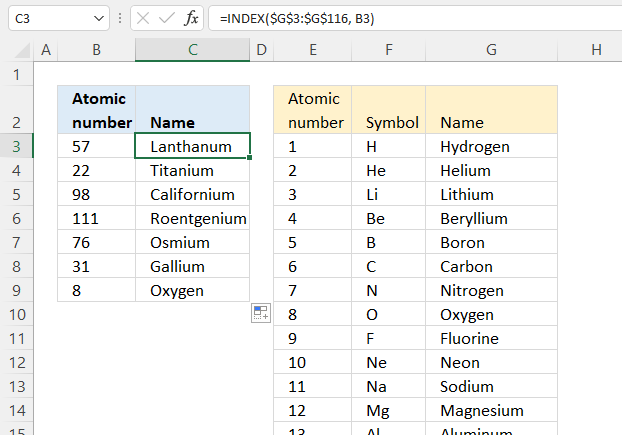The IFS function is great for smaller logical expressions but I recommend using a different formula combined with a table to avoid mega-formulas.

This example demonstrates this, the table in cell range E3:G116 describes the atomic numbers in the periodic table and the corresponding symbol and element name.

Creating a large IFS function for this specific example is possible, remember, you are allowed to use up to 127 pairs of logical expressions, however, I recommend putting all logical expressions and corresponding values in a table. This makes it easier to troubleshoot and examine the formula.

Formula in cell C3:

=INDEX(\$G\$3:\$G\$116, B3)

### Explaining formula

The INDEX function returns a value from a cell range, you specify which value based on a row and column number.

INDEX(array[row_num][column_num], [area_num])

INDEX(\$G\$3:\$G\$116, B3)

becomes

INDEX(\$G\$3:\$G\$116, 57)

and returns the value in cell E59 which is "Lanthanum".

\$G\$3:\$G\$116 is an absolute cell reference meaning it won't change when you copy cell C3 and paste it to the cells below.

B3 is a relative cell reference meaning it changes when you copy cell C3 and paste it to the cells below.